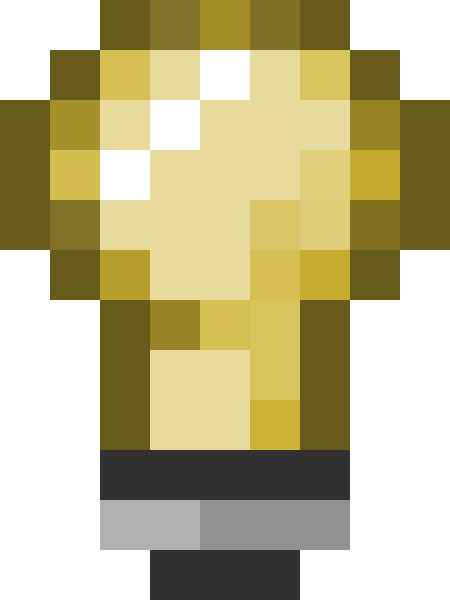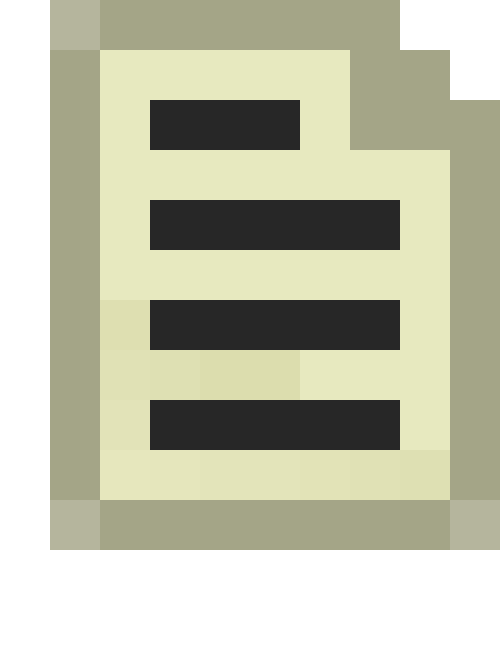# QAngle

(Redirected from Angles)

## Contents

QAngle is a C++ class that represents a three-dimensional Euler angle, offset from the cardinal Z axis. Each QAngle contains three vec_t rotation values:

• X pitch +down/-up
• Y yaw +left/-right
• Z roll +right/-left

`(-45,10,0)` means 45° up, 10° left and 0° roll.Tip:The axes are sometimes called rX, rY and rZ to distinguish them from vector axes.Note:Z is clamped to +/-50 for player viewangles.

## Declaration

```QAngle angMyAngle = QAngle(-45,10,0);
```
• You could also assign to the X, Y and Z member variables separately.
• The prefix `ang` (or sometimes just `a`) identifies the variable as an angle.

## Orientation

Confusingly, none of Source's rotation axes align with their position axes counterparts.

Even more confusingly, Hammer lists rotation axes as Y, Z, X! This is completely wrong, but luckily they are just labels: the values are stored in a single string (e.g. "-45 10 0") and interpreted by the game as rX rY rZ.

Remember that the effective direction of an angle is determined by its frame of reference. Typically, it will either be aligned to the world or to an entity.

## Converting from Vector

As there is no specific datadesc keyfield for angles, one chosen in Hammer will arrive as a vector. Its ordinates will represent degrees of rotation and not coordinates so it must be fixed up before use:

```QAngle angMyAngle = QAngle(vecFromHammer.x, vecFromHammer.y, vecFromHammer.z);

// Conversion back to a vector, if required
AngleVectors(angMyAngle, &vecFromHammer);
```